# Mixtures And Alligation

Mixture and alligation is an integral topic in the Quantitative section of the CAT exam. There are a lot of questions asked every year from this topic and thus, is very crucial from the examination point of view. So, here is an explanation of the Mixture and Alligation along with various illustrations to help the candidates learn the topic with ease.

Definitions of Mixture and Alligation

Here are some important definitions related to mixtures and alligation that would help to tackle the related questions with ease.

Mixtures

When two or more components are mixed in a certain ratio, a mixture is created.

Types of mixtures:

Simple Mixtures:- When two or more different ingredients are mixed together, a simple mixture is formed.

Compound Mixtures:- When two or more simple mixtures are mixed together, a compound mixture is formed.

Alligation:

To understand the concept of alligations, let’s take an example and look at it from all angles possible.

#### Watch The Below Videos for Alligation and Mixture:Example 1:

30 boys with an average weight of 60 kgs and 20 girls with an average weight of 40.Find the average weight of the whole class? Find the average weight of the whole class?

Solution:

To find the answer to the above question, one can use the formula for weighted average. Suppose the question was framed a bit differently, the Average weight of boys is 60, the average weight of girls is 40 and average weight of the whole class is 52.

Find the ratio between the number of boys and girls

Solution:

The fastest way to solve this question would be by using Alligation. Read below for its solution using alligation.

So what exactly is Alligation?

It is the reverse of weighted average; i.e. If the averages of two groups are separately given and the average of the whole group is given, then we can find out the ratio between the groups.

For example, in the above question, the data given is-

Average weight of boys=60, average weight of girls=40 and average weight of the whole class is =52

The data can be represented in an Alligation chart as given below and use it to find the ratio between the number of boys and girls in the class.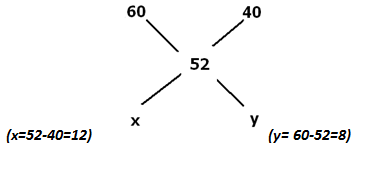Take the difference across: x= 60-52=8 and y= 52-40=12. That is how we get the ratio between the boys and girls as 12:8 or 3:2.Let us look at another question where you can use

Let’s look at another question where you can use Alligation.

Question:

In what ratio should a shopkeeper mix two types of rice, one costing 20 rupees/kg and another costing 10 rupees/kg to get a rice variety costing 14 rupees/kg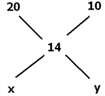Here also we can use Alligation as follows:

x=14-10=4 y=20-14=6 The ratio between the type 1 and type 2 rice is 4:6 or 2:3

Formulae In Mixtures & Alligations

There is no necessity to memorize all the formula given here, as all of them can be easily deduced with the help of logic.

Formula A,B and C are just algebraic representations of Alligation.

• (Quantity of cheaper entity )/(Quantity of expensive entity) = (Price of expensive entity – Mean price )/(Mean Price- Price of cheaper entity)
• Cost of Mixture(Cm)

Quantity of Component A =xa Quantity of Component B = xb Cost of Component A= Ca Cost of Component B= Cb

Then, Cm= (x_a C_a+x_(b ) C_(b ))/(x_a+ x_b )

• If you have 2 mixtures (M1 and M2), each having two components A and B

M_1 contains A & B in the ratio  p:q M_2 contains A & B in the ratio ? r:s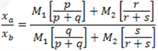Quantity of A and B in the final mixture (xa & xb)

• If a vessel contains “a” liters of liquid A; and ”b” liters are withdrawn and replaced by another liquid B of equal quantity and the operation is repeated n times, then:

1) (Liquid A left after nth operation)/(Initial quantity of A in the vessel) = (a-b)n/(a)n

2) (Liquid A left after nth operation )/(Liquid B left after nth operation) = ((a-b)n/(a)n )/(1- (a-b)n/(a)n )

Applications Of Mixtures

Consider the following common data to understand the application better.

A= 20 B=10 Na =30 Nb= 45 The answer will be 14, in each of the following cases.

1) Average of a class

Example:

Class A has 30 students scoring average marks of 20 and Class B has 45 students scoring average marks of 10.What is the average of both classes together (14).

2) Average price of goods

Example:

Sunita buys 30 kgs of sugar at Rs 20/kg and 45 kgs of sugar at 10/kg.

What is the average price? (Rs 14/kg).

3) Percentage

Example:

An antique article salesman makes a profit of 20% by selling 30% of his articles and a profit of 10% by selling 45% of his goods.

What is his net percentage profit? (14%).

4) Average Speed

Example:

A bus travels at 20kmph for 30 km and at 10 kmph for 45 km.

Find the average speed of the bus for the entire journey (14kmph).

5) Mixtures

A dishonest milkman dilutes two milk cans such that milk can A containing 30 liters is mixed with 20% water and milk can B containing 45 liters is mixed with 10% water. He combines both mixtures. Find the percentage of water in the final mixture (14%).

Application of Alligation

A) Application in profit & loss questions

Question: How many must kgs of rice costing Rs 8 per kg be mixed with 36 kg of rice costing Rs 5.40 per kg so that 20% gain may be obtained by selling the mixture at Rs 7.20 per kg?

a) 10 kg

b) 12 kg

c) 10.8 kg

d) 8 kg

Solution:

Always remember that we need to take only the cost price and not the selling price when we consider the price of all entities in the question.In the question, the selling price of the mixture is given. We need to find out the cost price using the profit percentage given=20%. Cost price × 1.2=7.2 , implies that cost price of the mixture is 6.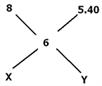From the Alligation chart,

X=6-5.40=0.6 Y=8-6=2

Thus the ratio between the quantity of type A rice and type B rice is 0.6:2 or 3:10 Therefore => x=10.8 kg.

B) Application in mixtures of liquids

Question: Vessel A contains milk and water in the ratio 4:5. Vessel B contains milk and water in the proportion 5:1.In what proportion should quantities be taken from A & B to form a mixture in which milk and water are in the ratio 5:4?

a) 2:5

b) 3:2

c) 2:3

d) 5:2

Solution:

For this question, let’s consider the proportion of milk in each mixture. In Vessel A, the proportion of milk in 4/((4+5) )=4/9.in vessel B, the proportion of milk is 5/(5+1) = 5/6.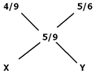The amount of milk in the mixture= 5/((5+4)) = 5/9

X=(5/6)-(5/9)=5/18

Y=(5/9)-(4/9)=1/9

The ratio is (5/18):(1/9)=5:2

C) Application in percentage questions

Question: A person has Rs.5000. He invests a part of it at 3% per annum and the remainder at 8% per annum simple interest. His total income in 3 years is Rs.750. Find the sum invested at different rates of interest?

a) 3500, 1500

b) 1000, 4000

c) 3000, 2000

d) 1800, 3200

Solution:

He is investing part of it at 3% at annum and remaining at 8% per annum Since he is getting Rs 750 as profit for 3 years, rate percent of the whole amount is 5%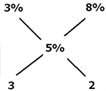That can be calculated from

PNR/100 = 750 ((5000 ×3 ×R))/100 = 750 R= 5%

From the Alligation chart,

The ratio of investment will be 3:2. Look at the answer options for that ratio.

Only option (c) (3000, 2000) gives that ratio.

Stay tuned with BYJU’S to get more lessons on various important CAT topics along with preparation tips and the latest CAT syllabus to strategize a proper preparation plan and ace the exam easily.